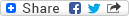#Two-Dimensional Mathematical Modelling of a Dam-Break Wave in a Narrow Steep Stream
ID Fortuna, Mateja (Avtor), ID Devjak, Tatjana (Mentor) Več o mentorju..., ID Krzyk, Mario (Avtor), ID Klasinc, Roman (Avtor), ID Četina, Matjaž (Avtor)PDF - Predstavitvena datoteka, prenos (1,05 MB)MD5: 56CE1824E5FE8CECCD779B35F21A0B50PID: 20.500.12556/rul/c73f5caf-36bc-4e50-9bb5-325bdd07faaa

Izvleček
The paper deals with hydraulic aspects of a wave, emerging as a result of a potential dam break of the upper storage reservoir of the pumpedstorage hydropower plant Kolarjev vrh. A two-dimensional depth-averaged mathematical approach was used. The upper storage reservoir and its dam failure were modelled with the mathematical model PCFLOW2D, which is based on the Cartesian coordinate numerical mesh. The results of PCFLOW2D were used as the upper boundary condition for the mathematical model PCFLOW2D-ORTHOCURVE, based on the orthogonal curvilinear numerical mesh. The model PCFLOW2D-ORTHOCURVE provided a tool for the analysis of flood wave flow in a steep, narrow and geometrically diversified stream channel. The classic Manning’s equation fails to give good results for streams with steep bed slopes and therefore, a different equation should be used. The application of the Rickenmann’s equation was chosen, presented in a form similar to Manning’s equation. For the purpose of the example given here, the equation was somewhat simplified and adapted to the data available. The roughness coefficient used at each calculation cell depended on the slope of that cell. The results of numerical calculations were compared to measurements carried out on a physical model in the scale of 1 : 200. Regarding the complexity of the flow phenomenon a rather good correlation of maximum depth was established: only at one gauge the difference in water depth was up to 27% while at the other four it was 7% of water depth on average.

Jezik: Neznan jezik dam-break wave, steep curved channels, two-dimensional mathematical model, orthogonal curvilinear coordinates, roughness coefficient, model PCFLOW2D-ORTHOCURVE Delo ni kategorizirano FGG - Fakulteta za gradbeništvo in geodezijo UL, Fakulteta za strojništvo, Aškerčeva 6, 1000 Ljubljana 2012 255-262 58 20.500.12556/RUL-684270039-2480 10.5545/sv-jme.2010.21610.07.2015 1862 931Kopiraj citatNazaj